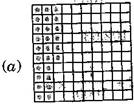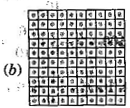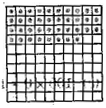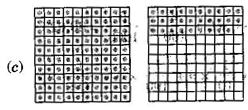### Decimals-Solutions Ex-8.2

CBSE Class –VI Mathematics
NCERT Solutions
Chaper 8 Decimals (Ex. 8.2)

Question 1.Complete the table with the help of these boxes and use decimals to write the number:Ones Tenths Hundreths Numbers (a)$\left(a\right)$ (b)$\left(b\right)$ (c)$\left(c\right)$
 Ones Tenths Hundreths Numbers (a)$\left(a\right)$ 0 2 6 0.26 (b)$\left(b\right)$ 1 3 8 1.38 (c)$\left(c\right)$ 1 2 9 1.29
Question 2. Write the numbers given in the following place value table in decimal form:
 Hundreds100 Tens10 Ones1 Tenths110$\frac{1}{10}$ Hundreths1100$\frac{1}{100}$ Thousandths11000$\frac{1}{1000}$ (a)$\left(a\right)$(b)$\left(b\right)$(c)$\left(c\right)$(d)$\left(d\right)$(e)$\left(e\right)$ ​01020 ​00311 ​32012 ​26092 ​53204 ​00521
Answer: (a) 0 x 100 + 0 x 10 + 3 x 1 + 2 x $\frac{1}{10}$ + 5 x $\frac{1}{100}$ + 0 x $\frac{1}{1000}$
= 0 + 0 + 3 + 0.2 + 0.05 + 0 = 3.25
(b) 1 x 100 + 0 x 10 + 2 x 1 + 6 x $\frac{1}{10}$ + 3 x $\frac{1}{100}$ + 0 x $\frac{1}{1000}$
= 1 + 0 + 2 + 0.6 + 0.03 + 0 = 102.63
(c) 0 x 100 + 3 x 10 + 0 x 1 + 0 x $\frac{1}{10}$ + 2 x $\frac{1}{100}$ + 5 x $\frac{1}{1000}$
= 0 + 30 + 0 + 0 + 0.02 + 0.005 = 30.025
(d) 2 x 100 + 1 x 10 + 1 x 1 + 9 x $\frac{1}{10}$ + 0 x $\frac{1}{100}$ + 2 x $\frac{1}{1000}$
= 200 + 10 + 1 + 0.9 + 0 + 0.002 = 211.902
(e) 0 x 100 + 1 x 10 + 2 x 1 + 2 x $\frac{1}{10}$ + 4 x $\frac{1}{100}$ + 1 x $\frac{1}{1000}$
0 + 10 + 2 + 0.2 + 0.04 + 0.001 = 12.241
Question 3. Write the following decimals in the place value table:
(a) 0.29
(b) 2.08
(c) 19.60
(d) 148.32
(e) 200.812
 Numbers Hundreds Tens Ones Tenths Hundreths Thousandths 100 10 1 110$\frac{1}{10}$ 1100$\frac{1}{100}$ 11000$\frac{1}{1000}$ (a) 0.29 0 0 0 2 9 0 (b) 2.08 0 0 2 0 8 0 (c) 19.60 0 1 9 6 0 0 (d) 148.32 1 4 8 3 2 0 (e) 200.812 2 0 0 8 1 2
Question 4. Write each of the following as decimals:
(a) $20+9+\frac{4}{10}+\frac{1}{100}$
(b) $137+\frac{5}{100}$
(c) $\frac{7}{10}+\frac{6}{100}+\frac{4}{1000}$
(d) $23+\frac{2}{10}+\frac{6}{1000}$
(e) $700+20+5+\frac{9}{100}$
Answer: (a) 20 + 9 + 0.4 + 0.01 = 29.41
(b) 137 + 0.05 = 137.05
(c) 0.7 + 0.06 + 0.004 = 0.764
(d) 23 + 0.2 + 0.006 = 23.206
(e) 700 + 20 + 5 + 0.09 = 725.09
Question 5. Write each of the following decimals in words:
(a) 0.03
(b) 1.20
(c) 108.56
(d) 10.07
(e) 0.032
(f) 5.008
Answer: (a) Zero point zero three
(b) One point two zero
(c) One hundred and eight point five six
(d) Ten point zero seven
(e) Zero point zero three two
(F) Five point zero zero eight
Question 6. Between which two numbers in tenths place on the number line does each of the given number lie?
(a) 0.06
(b) 0.45
(c) 0.19
(d) 0.66
(e) 0.92
(f) 0.57
Answer: All the numbers lie between 0 and 1.
(a) 0.06 is nearer to 0.1.
(b) 0.45 is nearer to 0.5.
(c) 0.19 is nearer to 0.2.
(d) 0.66 is nearer to 0.7.
(e) 0.92 is nearer to 0.9.
(f) 0.57 is nearer to 0.6.
Question 7. Write as fractions in lowest terms:
(a) 0.60
(b) 0.05
(c) 0.75
(d) 0.18
(e) 0.25
(f) 0.125
(g) 0.066
Answer: (a) 0.60 = $\frac{60}{100}=\frac{3}{5}$
(b) 0.05 = $\frac{5}{100}=\frac{1}{20}$
(c) 0.75 = $\frac{75}{100}=\frac{3}{4}$
(d) 0.18 = $\frac{18}{100}=\frac{9}{50}$
(e) 0.25 = $\frac{25}{100}=\frac{1}{4}$
(f) 0.125 = $\frac{125}{1000}=\frac{1}{8}$
(g) 0.066 =